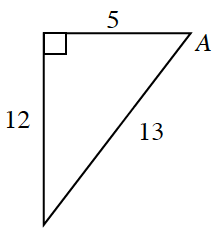Home > CCG > Chapter 5 > Lesson 5.1.3 > Problem5-30

5-30.

Use two different trig ratios to find the measure of $∠A$. Did you get the same answer both ways? Homework Help ✎Review the Math Notes box in Lesson 5.1.2.
With three known side lengths does it matter which trig ratio you use?

Remember when finding an angle measure you need to use inverse trigonometry.